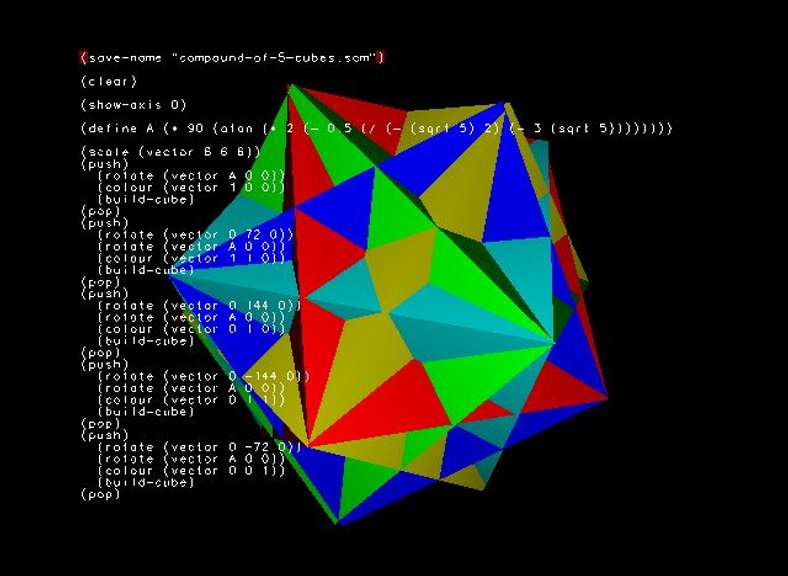# mathr / blog / #

## Five CubesText from E M Cundy and A P Rollett - Mathematical Models (Third Edition).

3.10.6. Five cubes in a dodecahedron

[Fig. 167]

The edges of the cubes lie by fives on the faces of the dodecahedron forming star-pentagons; each edge is thus divided by the two other edges in golden section.

The solid common to the cubes as a whole is bounded by thirty rhombi, one on each cube face, and is thus a rhombic triacontahedron.

The edges of these cubes can be grouped to form equilateral triangles, ordinary pentagons, and star pentagons, besides the squares which are the cube faces. By taking different combinations of these figures, several stellated Archimedean polyhedra can be formed. Full details can be found in Coxeter, op. cit., pp. 440-3.

The bibliography seems to indicate (non-definitively) that the referenced work is H S M Coxeter - Regular Polytopes.

Anyway, I had a go at implementing this solid in Fluxus, and after an hour of head-scratching and scribbling to figure out the trigonometry, this was the result:

(clear)

(define A (* 90 (atan (* 2 (- 0.5 (/ (- (sqrt 5) 2) (- 3 (sqrt 5))))))))

(scale (vector 5 5 5))
(push)
(rotate (vector 0 0 0))
(rotate (vector A 0 0))
(colour (vector 1 0 0))
(build-cube)
(pop)
(push)
(rotate (vector 0 72 0))
(rotate (vector A 0 0))
(colour (vector 1 1 0))
(build-cube)
(pop)
(push)
(rotate (vector 0 144 0))
(rotate (vector A 0 0))
(colour (vector 0 1 0))
(build-cube)
(pop)
(push)
(rotate (vector 0 -144 0))
(rotate (vector A 0 0))
(colour (vector 0 1 1))
(build-cube)
(pop)
(push)
(rotate (vector 0 -72 0))
(rotate (vector A 0 0))
(colour (vector 0 0 1))
(build-cube)
(pop)

EDIT: I later found out the equations I derived were wrong...